# Improving the Synchronization by Closed-Loop Fine-Timing Feedback¶

In the previous notebooks, we have relied on the Schmidl&Cox metric to perform the coarse timing estimation, whereas the fine timing (i.e. the residual timing error) was compensated by the channel equalization after according channel estimation.

We have seen, that in particular the interpolation in the complex plane becomes difficult, when the coarse timing is too much off. Hence, we added an extra phase-detrending algorithm for the channel estimation to overcome this issue. Though, in case the synchronization started the frame too late (i.e. after the CP had ended), samples were lost and the frame could not be fully equalized.

In this notebook, we will exploit the continuous signal structure to improve on the synchronization. More detailed, we consider the transmit signal to be a continuous stream of OFDM frames of length $N$. Hence, the receiver knows that every $N$ samples, a new frame should start. We will exploit this knowledge to improve the timing synchronization stability. In addition, by using a feedback loop between the channel estimation (i.e. the fine-timing estimation) and the S&C-unit, we will stabilize the synchronization as such, that it detects the frame start up to a fraction of a sample and hence does not produce too strong phase rotation in the frequency domain.

This notebook does not show the actual implementation of the algorithms. The principle is relatively simple, though the actual implementation is slightly involved due to the streaming mode of the chain. Consider to check the accompanying source code for details.

To start, let's import the common components from the library:

In :
# Source code has been redacted in online version
# Omitting 6 lines of source code


Also, we import OFDM functional blocks including the comb pilot transmitter, estimation and receiver blocks we implemented in the previous notebook:

In :
from audioComms.ofdm import OFDM
from audioComms.ofdm import CombPilotRandomDataOFDMTransmitter, CombPilotOFDMReceiver, CombPilotChannelEstimator
from audioComms.ofdm import CFOExtractor, CFOCorrection

from audioComms.synchronization import SchmidlCoxDetectPeaks, SchmidlCoxMetric


## A continuous frame structure¶

Before we actually exploit the frame structure, let us add one subtle modification: Up to now, we had inserted zeros between the OFDM frames to make the beginning of a frame visually recognizable:

In :
# Source code has been redacted in online version
# Omitting 8 lines of source code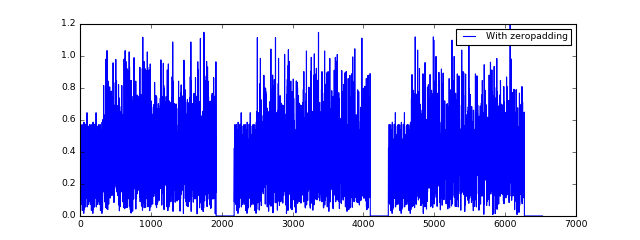We see, that between each OFDM frame there is a zero-value area. However, this vanishing region is misleading the receive-energy metric in the Schmidl&Cox calculation unit and can actually strongly distort the S&C metric. From now on, we will omit this extra guard region in favor of a more time-efficient and simpler transmission:

In :
tx_nozeros = CombPilotRandomDataOFDMTransmitter(environment=None, ofdm=ofdm,
insertZeropadding=False)  # Note this extra parameter

s_nozeros = np.hstack([tx_nozeros._generateSignal() for _ in range(3)])

plt.figure(figsize=(8,3));
plt.legend(loc='best');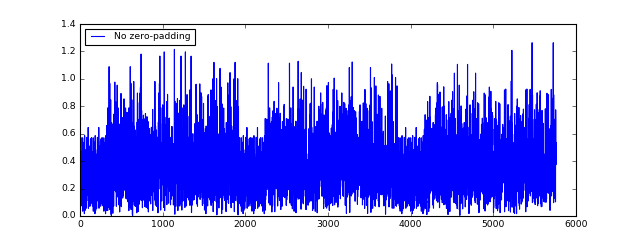Thanks to the extra parameter insertZeroPadding, the transmitted signal does not contain the zeros anymore.

In a first investigation, we will simply use the available objects and count the samples between the estimation of a frame start. The expected distance between frames is given by the frame length itself, which is:

In :
frameLen = (ofdm.K+ofdm.CP)*(1+ofdm.Npayload)
print (frameLen)

1920


This equation holds, as one OFDM symbol contains $K+CP$ samples, and we have Npayload payload symbols and 1 preamble. The extra information the receiver has and we will exploit is the following:

There is a frame every 1920 samples.

However, the above statement only holds, when there are no buffer underruns or otherwise samples lost on the audio channel. Hence, we still need to adapt the synchronization to changing signal conditions. Let's look later on how this can be achieved.

First, the following function plots the amount of samples between the detection of the frame start over time. Ignore the useStreamingMode parameter for now.

In :
%%tikz -s 800,300 -l positioning,arrows,automata
\input{tex/08-01}In :
# Source code has been redacted in online version
# Omitting 44 lines of source code


We define a common OFDM setting used for the following experiments:

In :
ofdm = OFDM(K=256, Kon=201, CP=64, Npayload=5, qam=4, pilotSpacing=10)


Now, let's run our default synchronization and see, how many samples apart the detected frames are. Here, we insert some noise and multipath effect to make the detection more difficult:

In :
# Source code has been redacted in online version
# Omitting 7 lines of source code

Expected distance between frames: 1920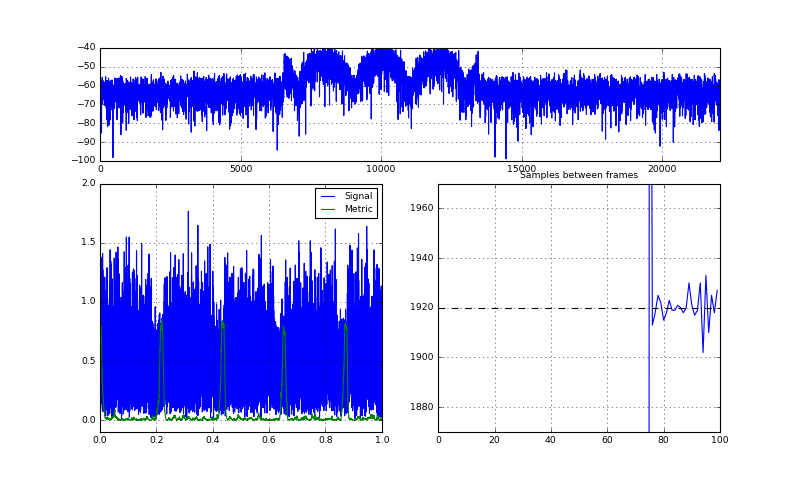Stop received from external


If the synchronization would have always found the same frame start, the number of samples between the frame start would have been constant (since each frame has the same length). However, as we see, the measurement varies, indicating that the S&C metric does not detect the same start sample in every frame. This problem is mainly due to the noise. As we have seen before, changing the frame start imposes more calculations at the channel estimation side, and hence should be avoided. Moreover, given that the synchronization can only be considered successful, if the frame start is detected within the CP, a shorter CP makes the synchronization even more difficult. On the other hand, a shorter CP implies larger spectral efficiency, so we should strive to shorten it as much as possible.

For now, let's see, if the same thing happens in the audio channel:

In :
# Source code has been redacted in online version
# Omitting 4 lines of source code

Expected distance between frames: 1920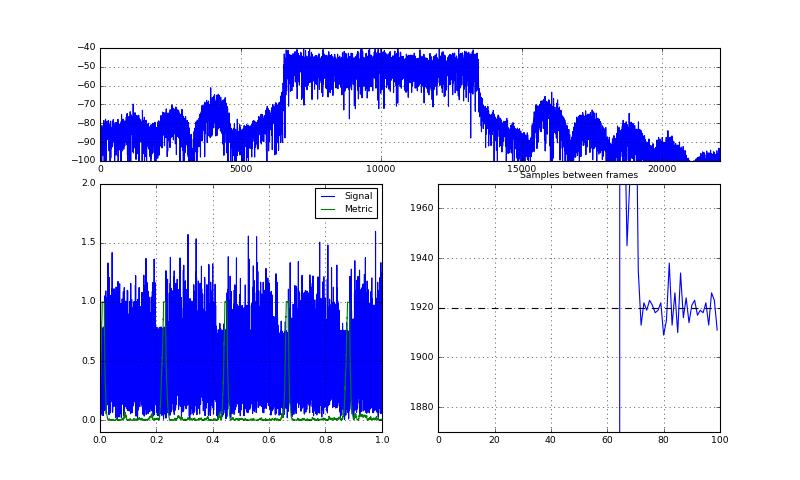Stop received from external
Stream Stopped 1
Stream Stopped 2


Obviously, also the audio channel obeys the problem: The number of samples between each frame changes, hence the coarse S&C synchronization does not reliably detect the start of the frame. How can we overcome this problem? The next section is dedicated to a simple method:

## The Synchronization State Machine¶

To improve the synchronization behaviour, we shall make use of the knowledge of the frame duration. However, using this knowledge exclusively would detach us from the actual S&C metric. Hence, we need to find a way to combine both information: The S&C metric and the knowledge of the frame duration.

The simplest method is to use a state machine: In the startign state, we need to estimate an initial starting point of the frame. As soon as we would have found one frame, we switch to the streaming mode: We could cut the received signal every 1920 samples and produce a new frame. Though, to make sure it still fits to the S&c metric, we need to check if the S&C metric indicates a peak where we expect it. If the S&C metric is high where we expect it, we are assured that a frame is indeed contained in the received signal and we remain in the streaming state. On the other hand, if the metric does not go above the threshold, we should switch back to the initial state and start again with a coarse synchronization:

In :
%%tikz -l arrows,automata,positioning -s 800,300
\input{tex/08-syncstatemachine}In above function's code, we had already added a parameter useStreamingMode which switches the S&C peak detection unit to use the proposed state machine above. Let us try out the code:

In :
runTransmissionCountSamplesBetweenFrames(ofdm,
lambda env, ofdm: CombPilotRandomDataOFDMTransmitter(env, ofdm, insertZeropadding=False),
AudioChannel,
B=441*5*4, Fc=10000,
useStreamingMode=True)  # switch on the streaming mode

Expected distance between frames: 1920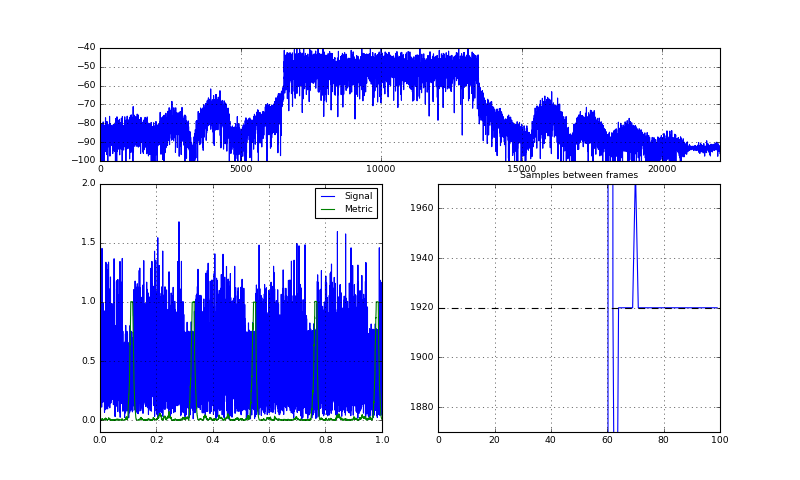Initial sync establibhed
Initial sync establibhed
Underrun!
Initial sync establibhed


Great! Now, as long as the Rx level is strong enough (and the S&C metric is hence reasonably high) the number of samples between the frames is constant. If the signal fades away (or there is a buffer underrun) such that a frame is lost, the initial peak finding state is executed.

Let's look at what happens with a subsequent OFDM demodulation:

In :
%%tikz -l positioning,arrows,automata -s 800,300
\input{tex/08-02}We add the comb-type pilot OFDM receiver to the chain and let's look at the results. You can ignore the parameter withTimingCorrection for now.

In :
def runTransmissionWithWithOFDMDemodulation(ofdm, transmitObjFunc, channelFunc, rxObjFunc, B=441*5*4, Fc=8000, useStreamingMode=True, withTimingCorrection=False):
frameLen = getattr(ofdm, 'frameLen', (ofdm.K+ofdm.CP) * (1+ofdm.Npayload))  # SC premble + payload

# Create the components
env = Environment(samplerate=44100)

# transmitter
tx = transmitObjFunc(env, ofdm)
# The number of samples between each frame start is the length of one signal vector from the transmitter
expectedTimeBetweenFrames = len(tx._generateSignal())

# channel
chan = PassbandChannel(env, channelFunc=channelFunc, Fc=Fc, B=B, cfo=0)

# synchronization
scMetric = SchmidlCoxMetric(env, ofdm=ofdm, minEnergy=1)
scDetect = SchmidlCoxDetectPeaks(env, K=ofdm.K, CP=ofdm.CP, frameLen=frameLen)
if useStreamingMode:
scDetect.assumeStreamingMode(expectedTimeBetweenFrames)
cfoExtract = CFOExtractor(env, ofdm, rate=B)
cfoCorrect = CFOCorrection(env, rate=B)

rx = rxObjFunc(env, ofdm)

# visualization
fig = env.figure(figsize=(10,6))
gs = GridSpec(3,2)
showMetric = PlotWaveform(env, windowDuration=1, rate=B, axes=fig.add_subplot(gs[0,0]), numLines=2, ylim=(-0.1,2), signalTransform=abs)

showTimeBetweenFrames = PlotWaveform(env, axes=timeAxis, integerXaxis=True, windowDuration=100, ylim=(expectedTimeBetweenFrames-50,expectedTimeBetweenFrames+50), title='Samples between frames')
timeAxis.axhline(expectedTimeBetweenFrames, color='k', ls='--') # mark the expected value

showHest = PlotBlock(env, axes=fig.add_subplot(gs[0,1]), numLines=2, ylim=(-4,4), title='Channel Estimation')
showConstellation = PlotConstellation(env, axes=fig.add_subplot(gs[1:,1]), title='Rx Constellation', numLines=5, xlim=(-1.5,1.5))

if withTimingCorrection:
showTimingCorrection = PlotWaveform(env, windowDuration=20, integerXaxis=True, axes=fig.add_subplot(gs[2,0]), title='Timing correction', ylim=(-2.5,2.5))

# set up the connections
tx.transmitsTo(chan)
chan.transmitsTo(scMetric)

scMetric.transmitsTo(scDetect)
scMetric.transmitsTo(showMetric)

scDetect.transmitsTo(cfoExtract, stream='P_metric')
scDetect.transmitsTo(cfoCorrect, stream='frame')
scDetect.transmitsTo(showTimeBetweenFrames, stream='samplesBetweenFrames')

cfoExtract.transmitsTo(cfoCorrect, stream='CFO')

cfoCorrect.transmitsTo(rx, stream='frame')

rx.transmitsTo(showHest, stream='Hest')
rx.transmitsTo(showConstellation, stream='constellation')
if withTimingCorrection:
rx.transmitsTo(scDetect, stream='timingCorrection')
rx.transmitsTo(showTimingCorrection, stream='timingCorrection')

env.run()


First, let's run the new synchronization in the simulated channel:

In :
# Source code has been redacted in online version
# Omitting 8 lines of source code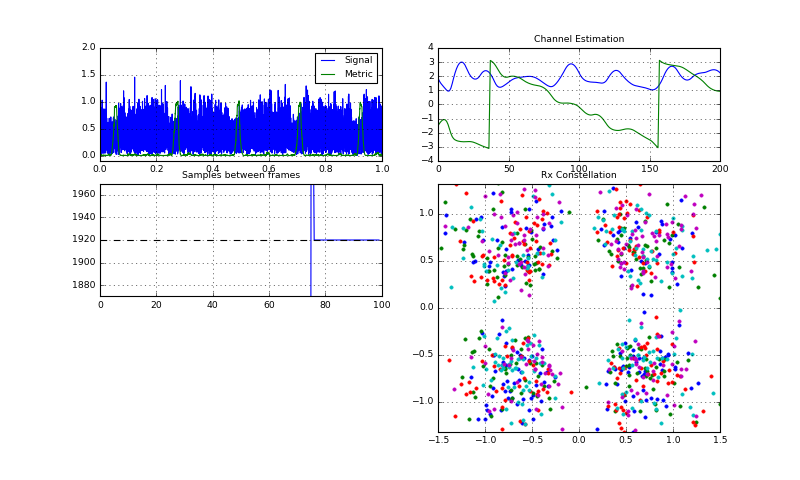Initial sync establibhed


Looking at the channel estimate, the number of phase wrap-arounds remains almost constant for each frame, which indicates that always the same frame start was detected. However, also a problem already arises here: If it happens that the initial synchronization point was pretty bad (i.e. few samples off the actual frame start), we have a lot of phase wrap arounds in the channel estimate and hence the interpolation becomes bad (Note that we do not use the phase-detrending interpolation technique here). Even worse, if it happens that the synchronization point was found too late, i.e. after the CP we even lose samples. Due to the streaming mode, this error will never be recovered from unless, a frame is completely lost and the sync switches back to the initial state.

We can see the same effect with the audio channel:

In :
# Source code has been redacted in online version
# Omitting 5 lines of source code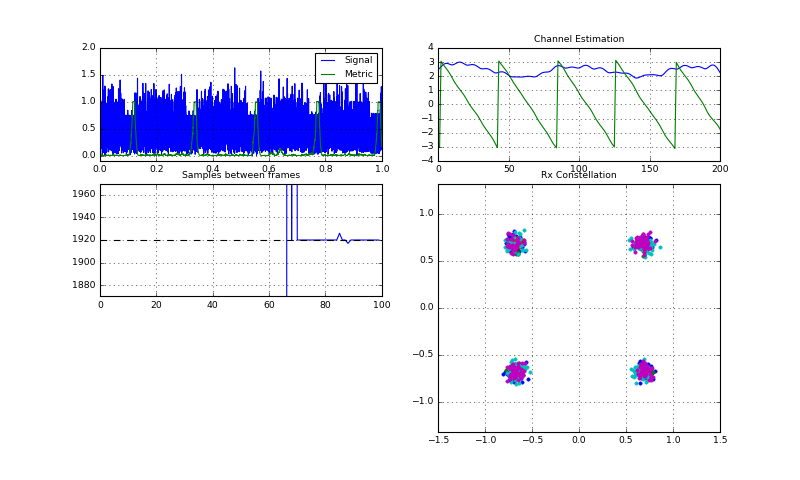Initial sync establibhed
Initial sync establibhed
Underrun!
Underrun!
Initial sync establibhed
Initial sync establibhed
Stream Stopped 1
Stream Stopped 2


With each restart of the chain above, a different synchronization point is found, but this point is then kept until the signal is faded away and a new fresh synchronization is performed.

Hence, despite the current methods keeps the synchronization stable, it also keeps the synchronization error stable. Hence, an initial synchronization error is kept forever. In the following section, we will see how we can overcome this problem:

## A closed control loop for fine-timing correction¶

The idea for solving the problem of the remaining and constant error due to the streaming is the following:

At the channel estimation unit, we have the information about the fine-timing error (the number of phase wrap arounds of the channel estimate). If we feedback this information to the synchronization unit, we can adapt the detection of the frame start and eventually converge to a stable synchronization that starts the frame at the correct timing. We form a closed control loop between the synchronization and the modulator. Without going deeply into the theory of these control loops, we just use some parameters that work to get the loop stable and working.

In :
%%tikz -l positioning,arrows,automata -s 800,300
\input{tex/08-03}